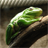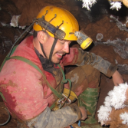# Determining probability on an arc

563
1
08-01-2011 10:33 AMby
New Contributor
Hi,

I am trying to determine the probability of finding targets on an arc using a probability raster that I have created. Basically there are two components to the project. I created a probability raster dataset by performing a weighted overlay of the slope of the terrain and the population per square mile of the area that I am working with. I then assigned a scale from a 0% chance to an 80% chance with increments of 10%. Each pixel in the raster dataset has its own probability (0, 10...-80%). I have several shapefiles of roads that lie on top of the raster layer. My goal is to determine the probability of finding someone traveling on an arc by calculating the number of pixels the arc runs through. So for example, if an arc runs through 10 pixels, 8 pixels have a value of a 50% chance and 2 pixels have a 80% chance.
50 * .8 + 80 * .2 = 56%. I need help repeating this process for every arc in the road shapefile I have. I would really appreciate it if anyone had an idea or knows a tool that will help me with this.

Many thanks,
Ryan
Tags (3)by